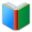# List 1 for 6th Grade MATH

Middle School Words: List 1 for 6th Grade MATH, all double sided cards of the list are in one page. You can download and print the worksheet from your browser directly.
 Free Online Vocabulary TestK12, SAT, GRE, IELTS, TOEFL
 Actions upon current list

 All lists of current grdae
Spelling Words:

Arts Words:

Social Words:

Math Words:

Science Words:

Literature Words:The SwineherdThe Red ShoesA Study In Scarlet
 Worksheet - Middle School Words: List 1 for 6th Grade MATH

 Print: List 1 for 6th Grade MATH

Exam Word - https://www.examword.com/

Middle School Words: List 1 for 6th Grade MATH

 base number the number that is going to be raised to a power
 circumference boundary line of a circle, figure, area, or object
 complement complete; consummate; make perfect
 convert change something into another form; transform
 coordinate plane coordinate system that specifies each point uniquely in a plane by a pair of numerical coordinates
 diameter length of straight line passing through the center of a circle and connecting two points on the circumference
 evaluate judge; examine and judge carefully; appraise
 factorization the resolution of an entity into factors such that when multiplied together they give the original entity
 non-terminating decimal a decimal numeral that does not terminate in an infinite sequence
 numerical expression combines numbers, variables, or constants using operators
 pi the ratio of a circle's circumference to its diameter; equal to 3.14159265358979323846...
 plane figure a two-dimensional shape
 prime factor the prime factors of a quantity are all of the prime quantities that will exactly divide the given quantity
 radius right line drawn or extending from the center of a circle to the periphery
 reciprocal concerning each of two or more persons or things; exchangeable; interacting
 sequences the action of following in order; serial arrangement in which things follow in logical order or a recurrent pattern
 similarity the quality of being similar
 simplify make simple; make less complex; make clear by giving the explanation for
 square units unit used to measure area
 substitution the act of putting one thing or person in the place of another; an event in which one thing is substituted for another